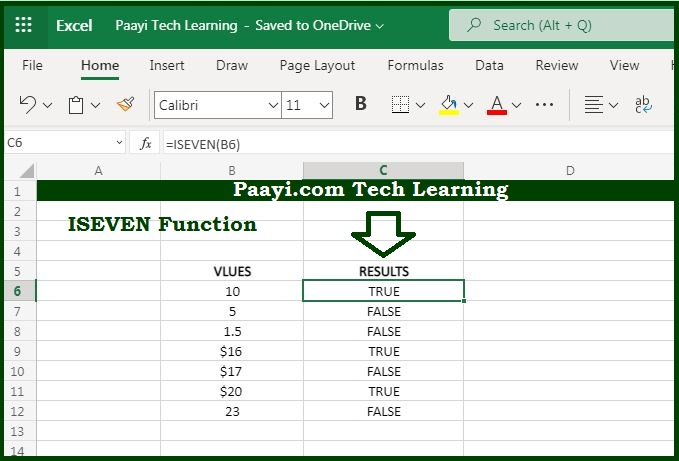# Learn How to Use Microsoft Excel ISEVEN Function

Written by | 0 Comments | 522 Views

In this article, you will learn how to use the Microsoft Excel ISEVEN function and its prime function in Microsoft Excel. You will also get to know the Microsoft Excel ISEVEN function return value and syntax with the help of some examples.

Microsoft Excel ISEVEN Function

The main function of the Microsoft Excel ISEVEN function is to test if the value is even or not. That means with the help of ISEVEN function you can able to get the return value as the TRUE if there is an even and FALSE for odd numbers. The ISEVEN function will return the #VALUE error in case the value is not numeric.

Return Value of ISEVEN Function

The return value will be the logical TRUE or FALSE.

Syntax of ISEVEN Function

=ISEVEN(value)

Where the arguments:

value: It is the numeric value to check.

## How to Use Microsoft Excel ISEVEN Function?You can use the ISEVEN function to check whether the value is even or not. ISEVEN function you can able to get the return value as the TRUE if there is an even and FALSE for odd numbers. The ISEVEN function will return the #VALUE error in case the value is not numeric. You can understand this in the picture shown above.

Usually, the value is provided as a cell address. ISEVEN function is the part of a functions group called the IS functions that all return the logical values TRUE or FALSE.